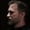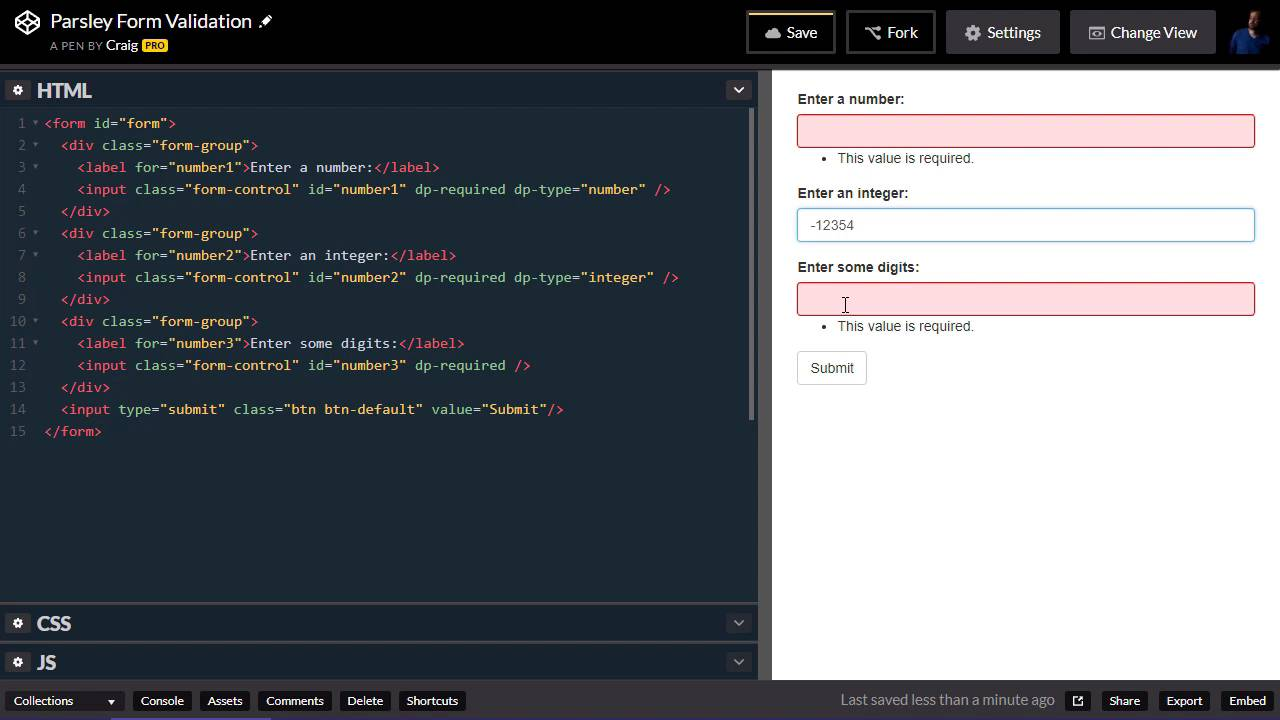FREELessons: 13Length: 53 minutes• Overview
• Transcript

# 2.8 Numeric Validations

In this lesson, you will learn how to use Parsley to perform various numeric validations for input text fields.

## 3.Conclusion1 lesson, 00:41

### 2.8 Numeric Validations

Hello, and welcome back. In this lesson, we're gonna take a look at validating numeric values using Parsley. And as you can see, we're starting with a whole different form here. It's set up the same way as our other one. I've added a little bit of CSS, and we've activated it with Javascript, still using that same dp namespace, so we don't have to type as much. And as you can see, I've set all of these up as required, and the reason I've done that is because it just makes it easier to test the values. Because we can just hit Submit, and then the live validation if you will, kicks in. So that we can start typing in and see as we type whether the value we enter is valid. Okay, so our first input field here says, Enter a number. And first of all you can find the URL for this starting pen in your course notes for this lesson and let's go ahead and fork it to create a new copy. And we'll get started with that new copy. So, our very first one says to Enter a number. Second one says to Enter an integer, the third one says to Enter some digits. And each one of these is handled, or validated in a different way. So let's take a look at the number first. So, there are a couple of ways that we could trigger this particular piece of validation. We can do it by changing our input here, giving it a type of number and we'll see what happens when we do that. So, let's go ahead and submit and then let's start typing in a number. So if we type in the number 1, everything looks fine. If we do 1.2 it says, this value seems to be invalid. Now 1.2 is a number, but when Parsley validates a number field with a type of number, it bases that on whatever the step value is by default, for that particular item. And by default in HTML, the step value is one. So, if this isn't a multiple of one, then it's not going to validate it properly. So I don't really like this particular method of validating a number. What I do like, is using the Parsley specific attribute. So instead of just giving this input a type of number, we're going to give it an attribute called dp-type or data Parsley type, if you're not using the custom namespace. But dp-type and we're gonna set that equal to number. And this is going to handle it in a little bit of a different fashion. So now, let's go ahead and hit Submit to trigger that validation. And now when we enter 1.2, we see that it is a valid number. If we get rid of that 2 and it's just 1 dot, then that's not a valid number. We have to have something after the dot if we put a dot there. So 1.0 is a valid number. So this makes a little more sense when we're validating whether something is a number. So let's talk about the integer validation. With integer we're gonna do the exact same thing. We're gonna use dp-type. But this time we're gonna set the type equal to integer. So this is gonna be handled a little bit differently, as you might imagine. If we enter any integer here, it's going to validate just fine. But if we do .1 for example, it's not going to validate properly because it is not a valid integer. So if we get rid of the .1, it works fine. And notice we can also put a negative at the beginning and make it a negative number and it validates just fine there because it is a negative integer. And then for our third field, it just simply says to enter some digits. The way this is validated is a little bit different than integer or number. What this is gonna do, is it's gonna look at every single character that we enter in and if every single character is not a digit, then it will not validate. So as you can imagine, the way we trigger this validation is we type dp-type, or data Parsley type, and set that equal to digits. So, let's go and hit Submit to trigger those validations. And remember, we can enter in a negative number here, as an integer for the second field. But if we try to do that for this field down here, -123, you'll see that it is not valid, because it doesn't care whether this is a valid integer or a valid number. It cares that every single character in this input field is a number. So we can only have the characters zero through nine in this particular field if we're validating it for digits. So this might be a good one to use for Social Security Number or telephone number, or something like that. That way the user can only enter in actual digits. No negative signs or anything like that. And since we're talking about numbers, let's also talk about validating the range of a number. So we can set a minimum value, a maximum value, or a range for any type of numerical value. So let's do all of this on the number field. So we have dp-type = number, we're gonna check to make sure that it's the right type. But we're also going to do a minimum number here. Now again, you can use the HTML5 attribute min and set that equal to a value. Or we can use the data Parsley value. And I'm gonna use dp-min and set that equal to a value of 10. Let's say that we want a minimum number to be 10. So we're gonna enter in a number here, we type in the number 1 and it says, this value should be greater than or equal to 10, so we type in another digit there. And it's now at least 10, so it validates fine. We can even come in and type 9.9 and it's not greater than or equal to 10 so it's not gonna validate, but we change it to 19.9, it works fine. All right, so let's also set a maximum value here. So let's do dp-max and set that equal to a value of 20. And so now, if we type in a value of 21 and submit, it does not validate. If we change that to a value of 9, it still does not validate, if we go somewhere in between, such as 12, then it validates just fine. And keep in mind that those numbers are inclusive, so if we type in 20 or 10, it will still validate. And then as you can imagine, if we don't want two separate values, we can combine these together into one value and I'll do this on the second field here, using dp-range. And so this works the same way that it worked when we were checking for the length of a string before. You basically have a set of square brackets and then inside those square brackets we would put our minimum value inside, there we go. Our minimum value, a comma and our maximum value. So this would be the same thing as doing this dp-min equals 10 and dp-max equals 20. It just combines it into one attribute. So for our second value here, let's go and hit Submit to trigger our validation. If we type in a 9 it's not valid, 10 is valid. Anything between 10 and 20 is gonna be valid. 20 is still valid, 201 is not valid. So those are just a few different ways that we can perform some validations on numerical values using Parsley. Thank you for watching.

Back to the top